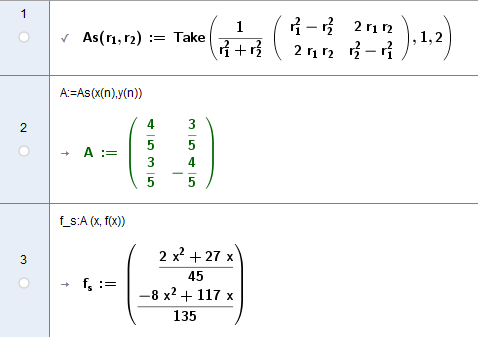# ApplyMatrix command to a 3d parametric curve

tush shared this question 3 years ago

Let c be a parametric curve in three-dimensions:

```c = Curve (
fx(t) , fy(t) , fz(t),
t , 0 , 1 )```

and M be a 3x3 matrix:

```M = {
{m11, m12, m13},
{m21, m22, m23},
{m31, m32, m33} }```

I want to plot the parametric curve c2 that is obtained by multiplying M with c.

ApplyMatrix command doesn't work in this case.

My work around at the moment is to define c2 to be

```c2 = Curve (
m11*x(c)+m12*y(c)+m13*z(c) ,
m21*x(c)+m22*y(c)+m23*z(c) ,
m31*x(c)+m32*y(c)+m33*z(c) ,
t , 0 , 1)```

which in other words means to calculate the product by hand.

(The matrix element M_ij is derived with Element(M,i,j), of course)

Is there a neater way to that?

Thanks a lot!try b = Curve((m1 a(t)), t, 0, 20) (warning: not b = Curve((m1 a), t, 0, 20))

like in attached

Files: foro.ggb1

`Is there a neater way to that?`
I don't think so. You could make a custom tool using that method though2

I have some similar

f(x)=1/2 x^2 + 1/3 x , n=(3,1)f_s was handelt as parametric function -10<=x<=10 - but I have no idea how to set the x limits. it is working only with x as function parameter!

So I made a Update-Script like

Execute({"fxy=Curve("+Element(f_p ,1)+","+Element( f_p,2)+", x, -100, 100)"})1

Thanks, I will try to elaborate your way a bit.Actually it kind of OK the way I mentioned at the original post, I just thought maybe there is a smarter way to do it, that I am not aware of.

BTW:

What is the meaning of ":=" is your first definition for 'As'?1

This CAS-Syntax definition of user functions (Keep Input to finish definition) or definition of variables.2

try b = Curve((m1 a(t)), t, 0, 20) (warning: not b = Curve((m1 a), t, 0, 20))

like in attached

Files: foro.ggb1

Simply Fantastic. Thanks very much indeed.2

it is useful for surface also